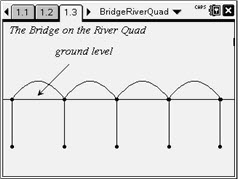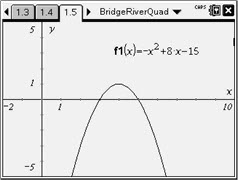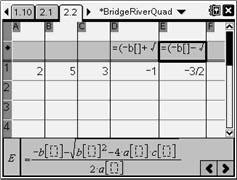# Activities

••• ##### Subject Area

• Math: Algebra I: Quadratic Functions

• ##### Author9-12

60 Minutes

• ##### Device
•TI-Nspire™ CX/CX II
•TI-Nspire™ CX CAS/CX II CAS
• TI-Nspire™
• TI-Nspire™ CAS
• ##### Software

TI-Nspire™
TI-Nspire™ CAS

2.1

• ##### Other Materials
By Lynne Plettenberg

## Bridge On The River Quad

#### Activity Overview

In the first part of this activity, students graph a quadratic function that models the shape of a bridge trestle. They then solve the related quadratic equation by completing the square, recording each step as they complete it. This list of steps is then generalized to deduce the quadratic formula. In the second part of the activity, students store the formula in their handhelds, compare its results with those of the nSolve command, and use it to solve several other quadratic equations.

#### Key Steps

•Pages 1.2 and 1.3 present the scenario of the problem: a bridge with four parabolic trestles. Students are given the equation y = –x2 + 8x – 15 as a model of the shape of the trestle and prompted to graph it on page 1.5.

•Students can work independently to complete the square for the equation –x2 + 8x – 15 = 0. Remind them that their first step must be to divide both sides of the equation by –1, in order to make the coefficient of x2 equal to 1.

The student worksheet provides a place for students to record their work as well as a description of each step.

•Finally, students use a spreadsheet to enter the coefficients a, b, and c and enter formulas for the positive and negative roots of the quadratic formula.